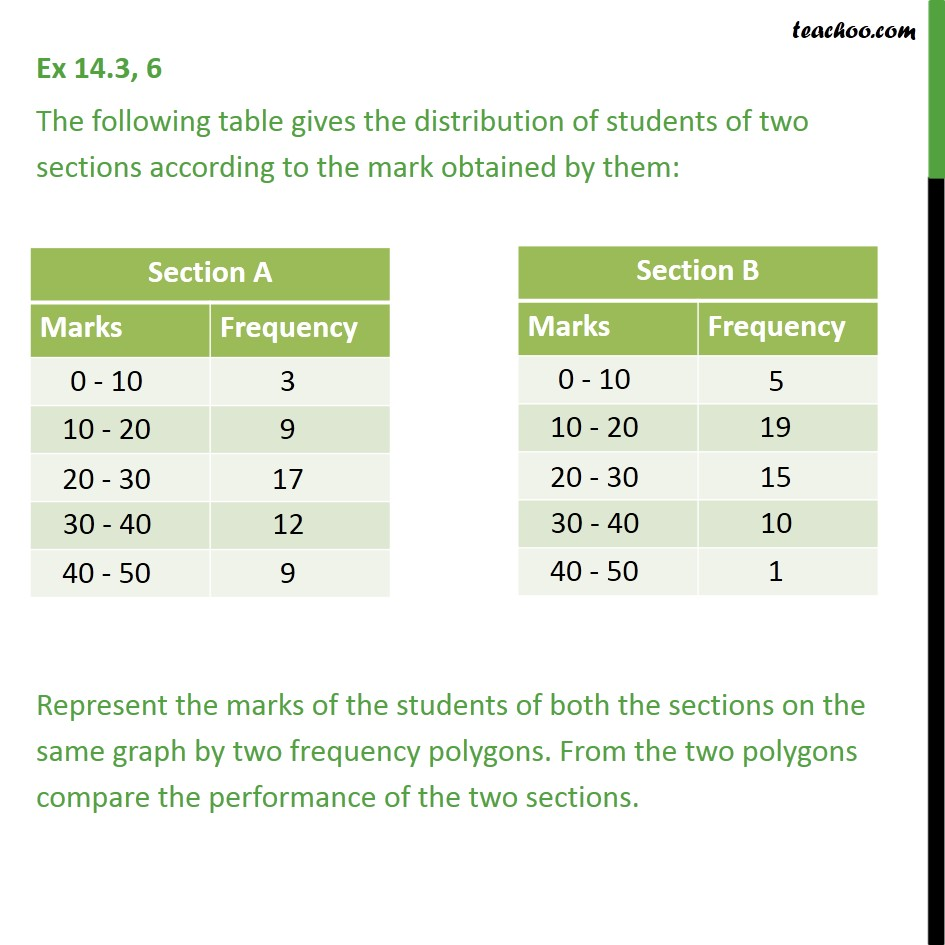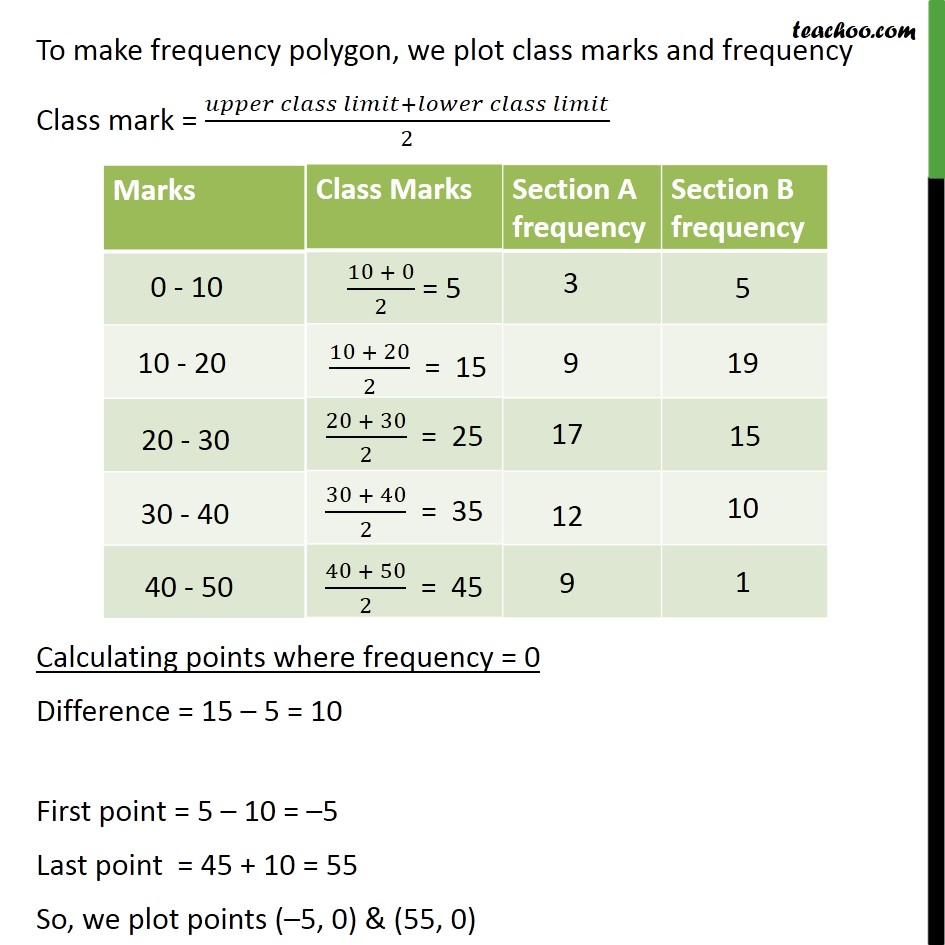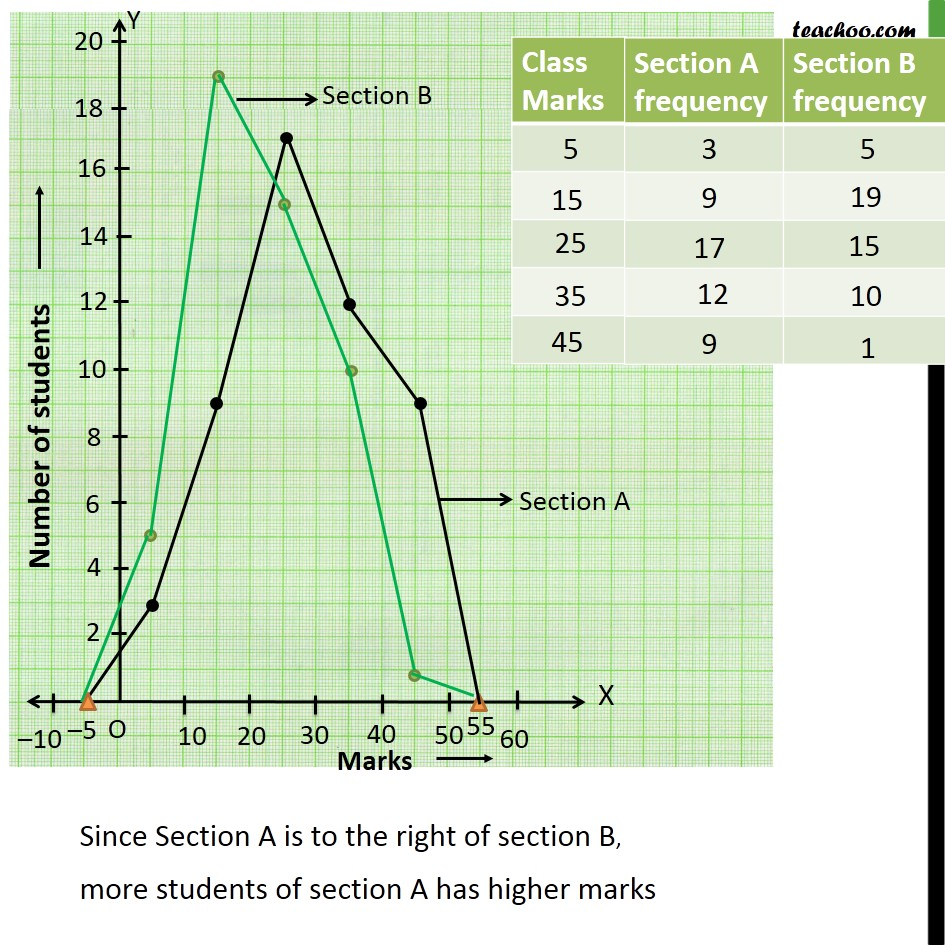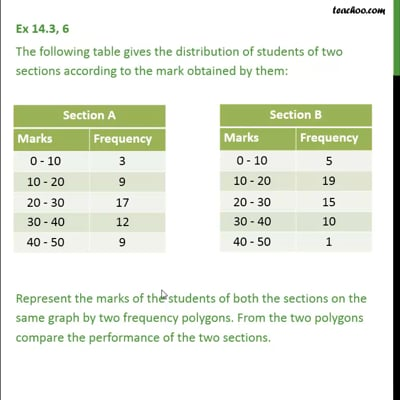Ex 14.3

Chapter 14 Class 9 Statistics
Serial order wiseThis video is only available for Teachoo black users

Solve all your doubts with Teachoo Black (new monthly pack available now!)

### Transcript

Ex 14.3, 6 The following table gives the distribution of students of two sections according to the mark obtained by them: Represent the marks of the students of both the sections on the same graph by two frequency polygons. From the two polygons compare the performance of the two sections. To make frequency polygon, we plot class marks and frequency Class mark = ( + )/2 Calculating points where frequency = 0 Difference = 15 5 = 10 First point = 5 10 = 5 Last point = 45 + 10 = 55 So, we plot points ( 5, 0) & (55, 0)